# Conduction: One Dimensional (Part - 5) Chemical Engineering Notes | EduRev

## Chemical Engineering : Conduction: One Dimensional (Part - 5) Chemical Engineering Notes | EduRev

The document Conduction: One Dimensional (Part - 5) Chemical Engineering Notes | EduRev is a part of the Chemical Engineering Course Heat Transfer for Engg..
All you need of Chemical Engineering at this link: Chemical Engineering

Conduction: One Dimensional, Heat Transfer

2.4 Heat conduction in bodies with heat sources
The cases considered so far have been those in which the heat conducting solid is free of internal heat generation. However, the situations where the internal heat is generated are very common cases in chemical industries for example, the exothermic reaction in the solid pallet of a catalyst.

We have learnt that how the Fourier equation is used for the steady-state heat conduction through the composites of three different geometries that were not having any heat source in it. However, the heat generation term would come into the picture for these geometries. It would not be always easier to remember and develop heat conduction relations for different standard and non-standard geometries. Therefore, at this point we should learn how to develop a general relation for the heat conduction that should be applicable to the entire situation such as steady-state, unsteady state, heat source, different geometry, heat conduction in different direction, etc. Again here we will consider that the solid is isotropic in nature, which means the thermal conductivity of the material is same in all the direction of heat flow.

To get such a general equation the differential form of the heat conduction equation is most important. For simplicity, we would consider an infinitesimal volume element in a Cartesian coordinate system. The dimensions of the infinitesimal volume element are d, d, and din the respective direction as shown in the fig.2.11.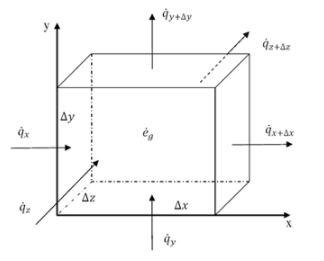Fig.2.11. Volume element for deriving general equation of heat conduction in cartesian coordinate

The fig.2.11 shows that the heat is entering into the volume element from three different faces of the volume element and leaving from the opposite face of the control element. The heat source within the volume element generates the volumetric energy at the rate of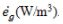According to Fourier’s law of heat conduction, the heat flowing into the volume element from the left (in the x-direction) can be written as,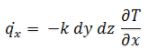The heat flow out from the right surface (in the x-direction) of the volume element can be obtained by Taylor series expansion of the above equation. As the volume element is of infinitesimal volume, we may retain only first two element of the Taylor series expansion with a reasonable approximation (truncating the higher order terms). Thus,

###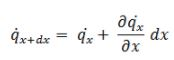or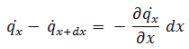The left side of the above equation represent the net heat flow in the x-direction. If we put the value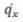of  in the right side of the above equation,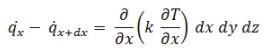In a similar way we can get the net heat flow in the y and z-directions,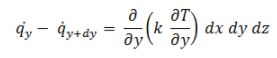and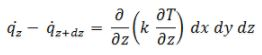As we know some heat is entering, some heat is leaving and some heat in generating in the volume element and as we have not considered any steady state assumption till now, thus because of all these phenomena some of the heat will be absorbed by the element. Thus the rate of change of heat energy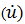within the volume element can be written as,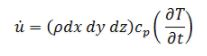where, cp is the specific heat capacity at constant pressure (J/(kg·K)), ρ is the density (kg/m3) of the material, and t is the time (s).

We know all the energy term related to the above problem, and with the help of energy conservation,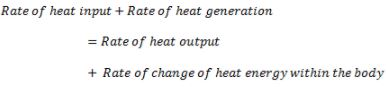On putting all the values in the above equation,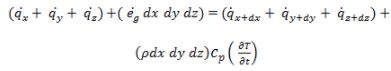or,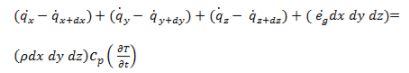or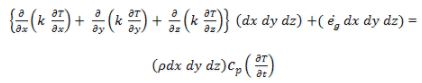or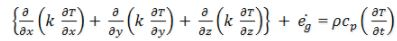As we have considered that the thermal conductivity of the solid is isotropic in nature, the above relation reduces to,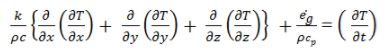or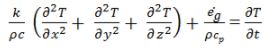or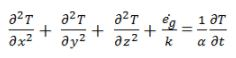or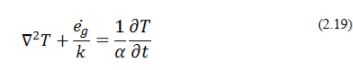where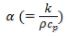is the thermal diffusivity of the material and its unit m2/s signifies the rate at which heat diffuses in to the medium during change in temperature with time. Thus, the higher value of the thermal diffusivity gives the idea of how fast the heat is conducting into the medium, whereas the low value of the thermal diffusivity shown that the heat is mostly absorbed by the material and comparatively less amount is transferred for the conduction. The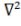called the Laplacian operator, and in Cartesian coordinate it is defined as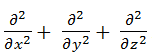Equation 2.19 is known as general heat conduction relation. When there is no heat generation term the eq.2.19 will become,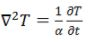(2.20)

and the equation is known as Fourier Field Equation.

Offer running on EduRev: Apply code STAYHOME200 to get INR 200 off on our premium plan EduRev Infinity!

## Heat Transfer for Engg.

46 videos|60 docs|59 tests

,

,

,

,

,

,

,

,

,

,

,

,

,

,

,

,

,

,

,

,

,

;